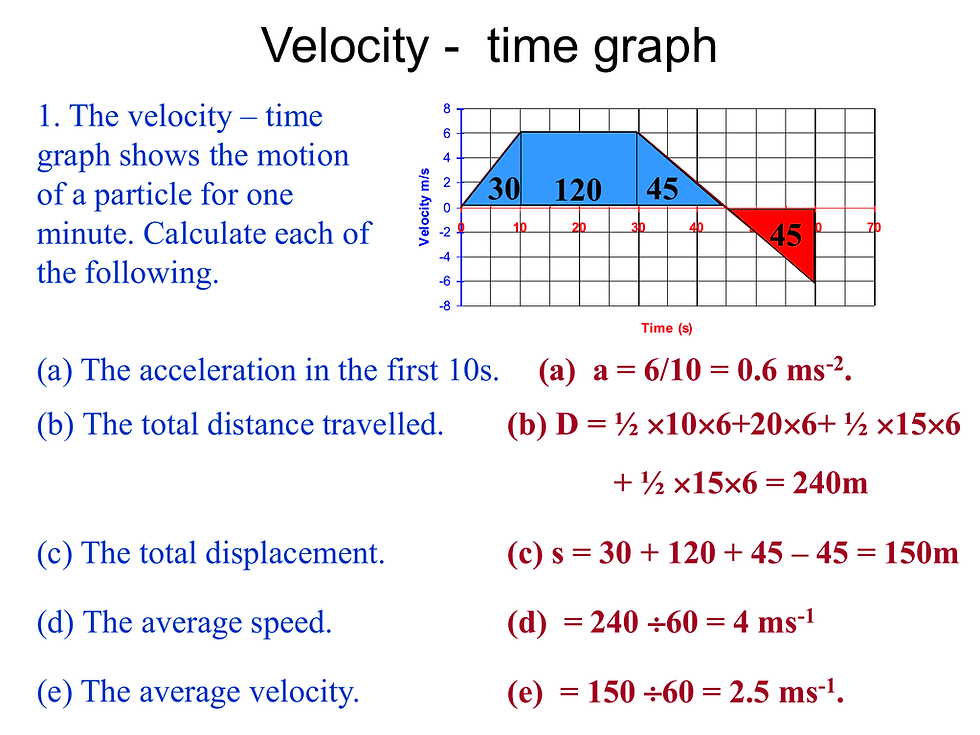top of page# SHARE

Mr Chen's anecdotes.

Search

# 3 GRAPHS YOU MUST KNOW TO ACE KINEMATICS

Updated: Apr 19, 2022

In the O level syllabus for physics, kinematics has always been a topic taught at the most initial stage. This is because one needs to fully understand and appreciate the effects of forces before they can explain the cause of these effects.

Most tutors would highlight the importance of kinematics as a prerequisite for subsequent chapters on classical mechanics. In addition, kinematics questions are usually presented along with a graph of various forms. It is therefore, imperative that a student fully comprehend the context and application of the graph. Below are the 3 graphs you will encounter in kinematics and the key features to be aware of.

(Follow @vantage_tutor on instagram to get video updates whenever a new article is released)

## Acceleration-Time Graph

Regardless of the graph given, it always comes down to 2 features. Gradient and area. Hence, a basic understanding of gradient and area and how to calculate them is required in order to excel.

## Displacement-Time GraphFamiliar looking I bet

Displacement-time graph is to help a student visualize the position of an object with reference to its origins as time passes by. Most of the time, students are asked to draw the displacement-time graph based on a velocity-time graph given. In such a graph, there is only 1 feature that can be used.Rise over Run

Recall from Secondary 2 Math that gradient is given by rise over run. In terms of formula, it is basically the difference between the 2 y-coordinates divided by the difference between the 2 corresponding x-coordinates.

When put into context of kinematics, the y-axis represents displacement while the x-axis represents time. Hence, gradient of a displacement time graph is effectively calculating for the velocity.

Back to Table of Content

## Velocity-Time GraphA typical kinematics question

Velocity-time graph is to help a student visualize the speed and direction of an object with reference to its origins as time passes by. This is one of the most commonly seen graph in kinematics questions. Very often, students are not only asked to calculate and quantify, but also explain the motion of the object in relevant terms. In such a graph, there are 2 features that are useful.

Similar to a displacement-time graph, the gradient is of relevance and this can be illustrated by applying the context of the axis to the formula for calculating gradient. Since the y-axis represents velocity while the x-axis represents time, the gradient is given by the difference between velocity at 2 time intervals divided by the time taken. Hence, gradient calculates for the rate of change of velocity, conveniently termed as acceleration.

### Area

In order to fully understand what the area under the velocity-time graph represents, students need to apply to context of the axis in conjunction with existing math concepts. Area is given by the product of perpendicular height and the length of sides.

Perpendicular height in the context of the graph is given by the y-axis which represents velocity. Whereas, the length of sides are given by the x-axis which represents time. Hence, when you calculate for area under the graph, you are effectively taking the product of velocity and the time taken. Ultimately, translating into calculation for displacement.

In most velocity time graph, a shape could be made out from the graph. Some students see a trapezium while others may see as separate entities of triangles and rectangles. Regardless, both will arrive at the same answer as long as the student is able to correctly identify the perpendicular height and length of the sides in the given shape.

Back to Table of Content

## Acceleration-Time GraphAcceleration-time graph is to help a student visualize the acceleration and direction of an object with reference to its origins as time passes by. In most exam papers, students are asked to sketch the acceleration-time graph based on the velocity-time graph given in the question. Hence, it is imperative that student understand acceleration to be the rate of change of velocity in order to sketch the graph.

### Area

Once again, apply the context of the y and x-axis to math. Y-axis represents the acceleration while x-axis represents the specific time interval. Perpendicular height in the context of the graph is given by the y-axis which represents acceleration. Whereas, the length of sides are given by the x-axis which represents time.

Hence, when you calculate for area under the graph, you are effectively taking the product of acceleration and the time taken. Ultimately, translating into calculation for velocity of object.

Back to Table of Content

## You're Not Alone

Singaporean students are known to be a tenacious bunch when it comes to examinations especially national ones like O level and N level. Most would want to grind it out on their own independently. However, few embark on the correct route for revision. Teachers are there for guidance but may not always be readily available.

"Should I drop Physics?"

We, at VANTAGE TUTOR, are known to guide students through chapters individually first before attempting questions that require an amalgamation of concepts from a variety of chapters. Our physics tutors pick out questions which test solely on one topic and we repeatedly attempt questions of similar nature in order to maximize your understanding. Only after you have mastered the individual concepts then we move on.

Precisely because of our experience in picking out such questions, we have not only learnt to compose a question on the spot, but also compiled a booklet of exam questions sorted out topically. You are welcomed to download the free O level physics question and notes bank sample today!

We are sure that you will benefit from the O level physics question package. You may contact us to purchase or to engage us as your private tutor. We look forward to hearing from you!